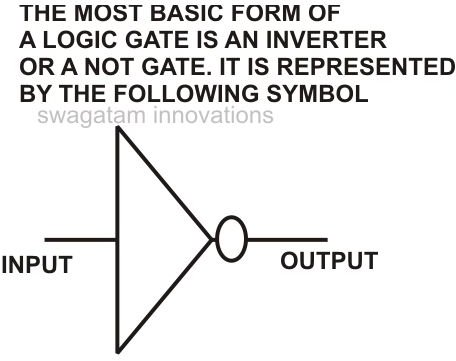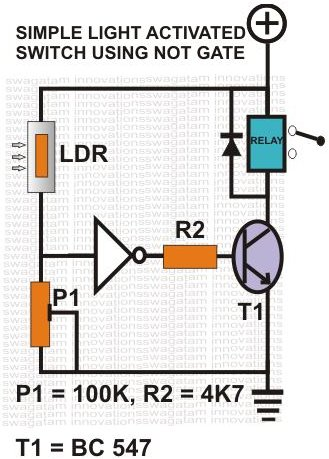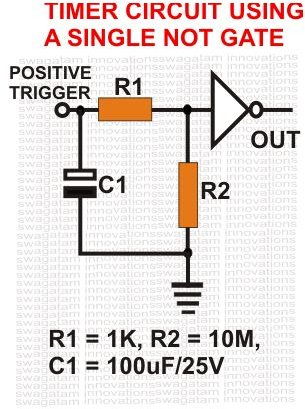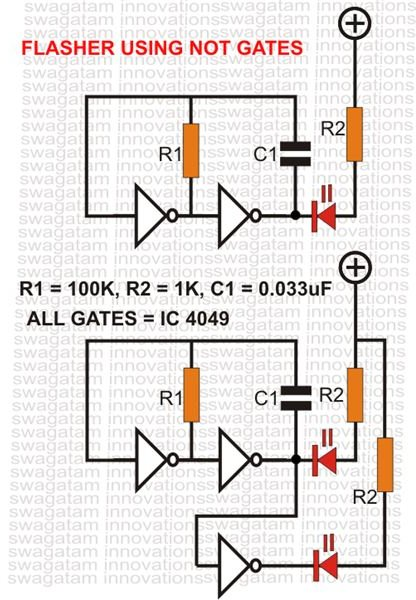How Digital Logic Gates Function

Introduction

The invention of CMOS digital logic gates has certainly proved to be the biggest possible leap that electronics could have taken. It totally changed the concept and the way electronics used to work prior to this invention. The rapid advancement in electronics that we are able to witness today is just because of this CMOS technology. Right from your cell phones and laptops to the satellites, all involve and depend on a network of logic gates.

A basic form of a CMOS IC may be discreetly made up of a single or many individually analyzing devices called the logic gates.

If we take human analogy and compare a computer to a human brain, then each logic gate involved in the computer circuit corresponds to each neuron present in our brain. Thus, a single CMOS logic gate may be considered as the basic building block of this technology and through intelligent combinations of these gates, complex and amazing results can be obtained, quite similar to the human brain.

Fundamental Characteristics of Logic GatesAs the name reveals, a CMOS gate, is a “gate” or a passage (to electrons) that may be opened or closed by providing certain well-defined input instructions. These instructions are specific to each type of gate and can be found through their Truth Tables.

The most important and identifiable feature of these gates is that they will not respond to any intermediate values or levels of voltages. Any voltage below 3 volts (approx.) will be taken as a zero voltage or logic “0” and anything above it, a positive or logical “1”. This is the only fundamental “language” that a logic gate understands, and that’s what makes them invariably accurate.

A typical CMOS gate basically comprises of an input and an output. The output is the function of its input and will respond to it as per its particular specifications. For example, the most preliminary form of such a gate is an “inverter” or the NOT gate. As the name suggests, the function of this particular gate is to invert or produce exactly the opposite result to that of its input status, i.e. if a positive voltage is applied to its input, the output will be a negative and vice versa.

Please note that the specific symbols of the various types of gates are just for recognizing purposes and for ease of their use in the schematics (nobody has cut through an IC to actually see how a gate looks), so don’t get confused by their symbolic appearances.

An IC may comprise many digital logic gates in one package (for example, the IC 4049 consists of 6 NOT gates), but in a schematic diagram these gates may be shown scattered all over, individually wired into separate circuits. Since all gates are identical, taking the help of the datasheet, you may just confirm the pin outs of the gates and connect them as shown in the particular schematic while constructing a circuit. You don’t have to bother regarding their serial number or placements.

Logic Circuit Design Project

Understanding a CMOS logic IC is perhaps more simple than understanding a transistor. Here we’ll take the example of the IC 4049 to show you how you can configure its logic gates (inverters) into practical circuits and make simple logic circuit design projects.Comparator: Referring to the along side diagram we see how a NOT gate may be wired into an accurate light sensor circuit. Here, its input is connected to a potential divider network made up of an LDR and a variable resistor or a preset P. As long as the light falling over the LDR does not produce a potential level greater than the set level of P1, the output of the gate remains stationary. But the moment the light level increases the set level and produces a voltage that’s recognizable as logic “1” by the input of the gate, its output immediately changes state and inverts to logic “0”.Timer: The next circuit shows how a NOT gate may be configured as a simple timer, perhaps the simplest and the most accurate short duration timer. Here we see, its input is connected to ground through resistor R2 and is held at logic “0”. Also, a capacitor C1 is attached to this input via resistor R1. At this stage its output will be obviously logic “1” (inverted). Now suppose we connect a positive voltage to the junction of R1 and C1. C1 instantly charges, the input is forced to logic “1” (because value of R2 is far greater than R1, therefore logic “0” is weaker) and the output becomes the opposite i.e. logic “0”.

Now what happens if the positive trigger is removed? The gate continues to sustain its position because of the stored charge in C1, until C1 completely discharges via R2 and the gate returns to its original position. The value of C1 and R2 determines the time period for which the gate remains latched.Flasher: This configuration shows (see figure) how simply by adding a capacitor and a resistor to a couple of NOT gates a beautiful LED flasher can be made. The rate of flashing can be adjusted or varied by changing the values of either the capacitor or the resistor. By adding another gate to its output you can convert it into an astable multivibrator producing alternate flashing of two LEDs. Now if you take the IC 4049, which contains 6 inverters in one pack, means you can construct three separate flashers or oscillators with different flashing rates and produce interesting flashing effects or results.

Precautions to be Observed While Working with CMOS Gates

Although the modern CMOS ICs have evolved into much more robust designs than what they were long time ago, still there are certain typical parameters these IC don’t like and due precaution needs to be taken to avoid them. They are as follows:

• Supply voltage should remain strictly within 5 to 15 volts DC.
• Input to the gates should never exceed the supply voltage.
• Supply terminals should be preferably (make it a practice) fitted with a decoupling capacitor.
• Inputs of unused or idle gates should ALWAYS be connected to a logic level (either positive or negative).

Once you grasp the basic rules regarding the functioning of logic gates as explained above, you should be able to build your own logic circuit design projects effortlessly.

Next time we will discuss regarding a few practical circuits using NAND gates.